# SPSS中如何对多个选项的结果进行计数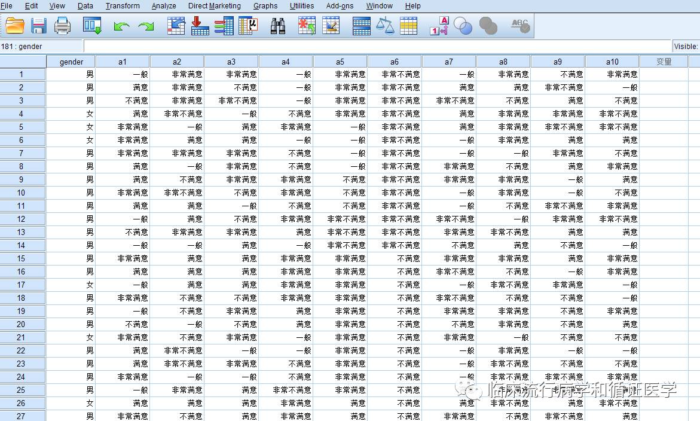但是首先需要计算的是每个研究对象10道题目中选择“满意”的题目有几题?这时候推荐使用SPSS转换功能下的计数功能，如下图：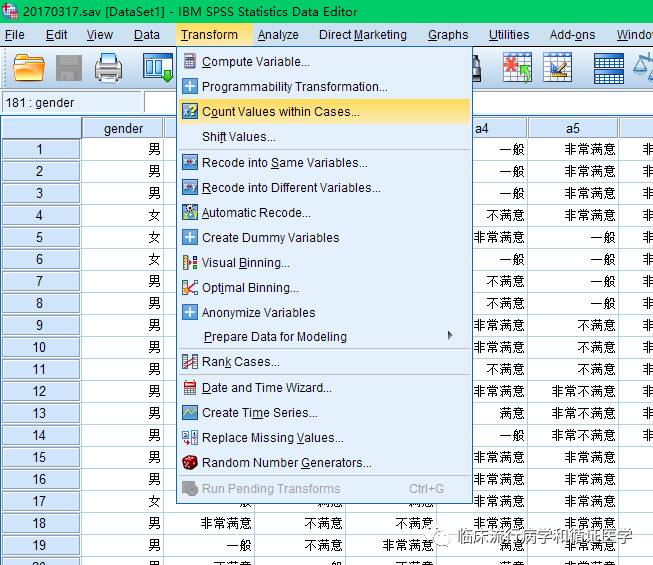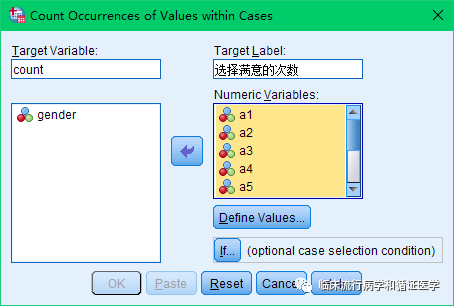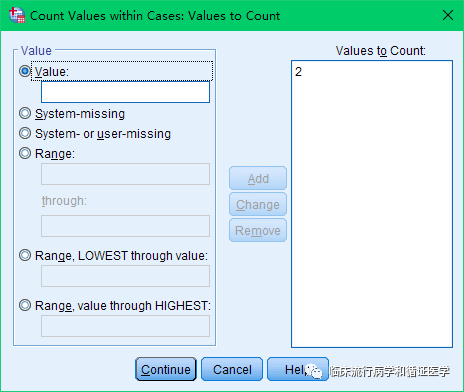然后点击OK，就会出现下图的结果，软件自动生存了count列，并在该列中展示了每个人选择“满意”的次数。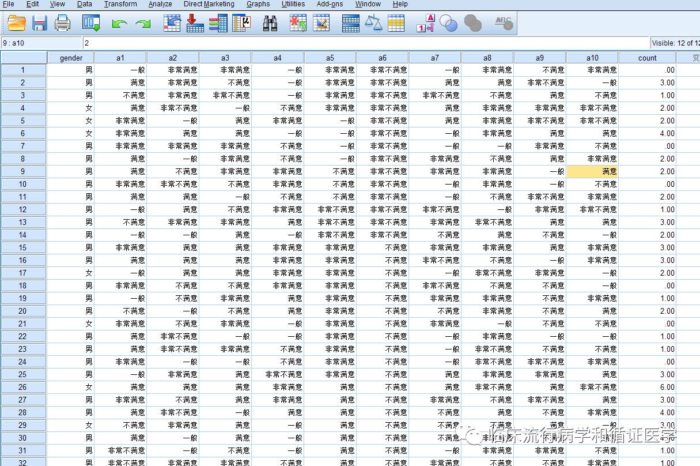这样的计算是不是比以前写程序来计算方便多了。另外这个计数功能还可以用在多个选项的计数上，比如求出每个研究对象“选择满意和非常满意”的次数。如下：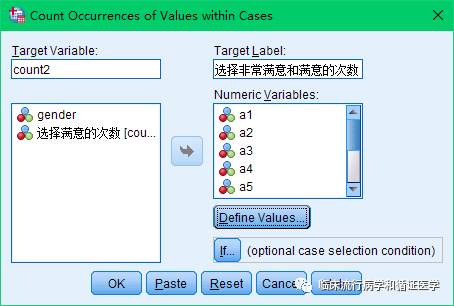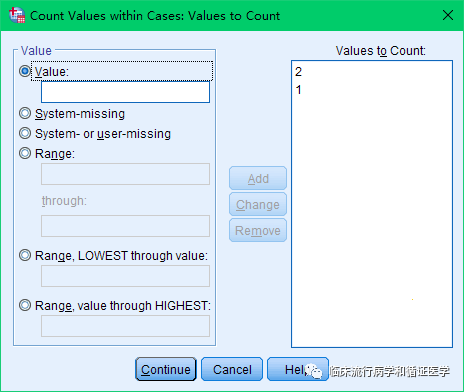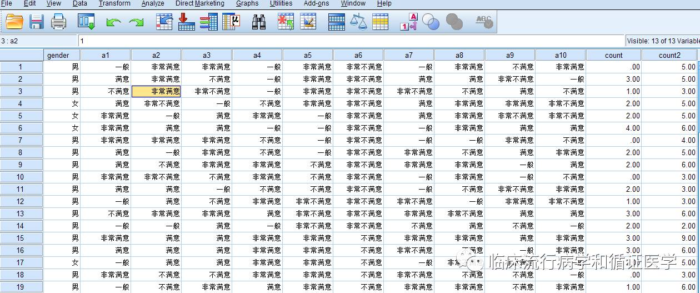呃，本文写到这儿就应该结束了。最后附上SPSS程序的另一种写法，让大家稍微感受一下两种写法的差异。

1、Count功能的语法：

COUNT count=a1 a2 a3 a4 a5 a6 a7 a8 a9 a10(2).

VARIABLE LABELS count '选择满意的次数'.

EXECUTE.

2、另一种语法：

if (a1=2) count=1.

if (a2=2) count=sum(count, 1).

if (a3=2) count=sum(count, 1).

if (a4=2) count=sum(count, 1).

if (a5=2) count=sum(count, 1).

if (a6=2) count=sum(count, 1).

if (a7=2) count=sum(count, 1).

if (a8=2) count=sum(count, 1).

if (a9=2) count=sum(count, 1).

if (a10=2) count=sum(count, 1).

VARIABLE LABELS count '选择满意的次数'.

EXECUTE.